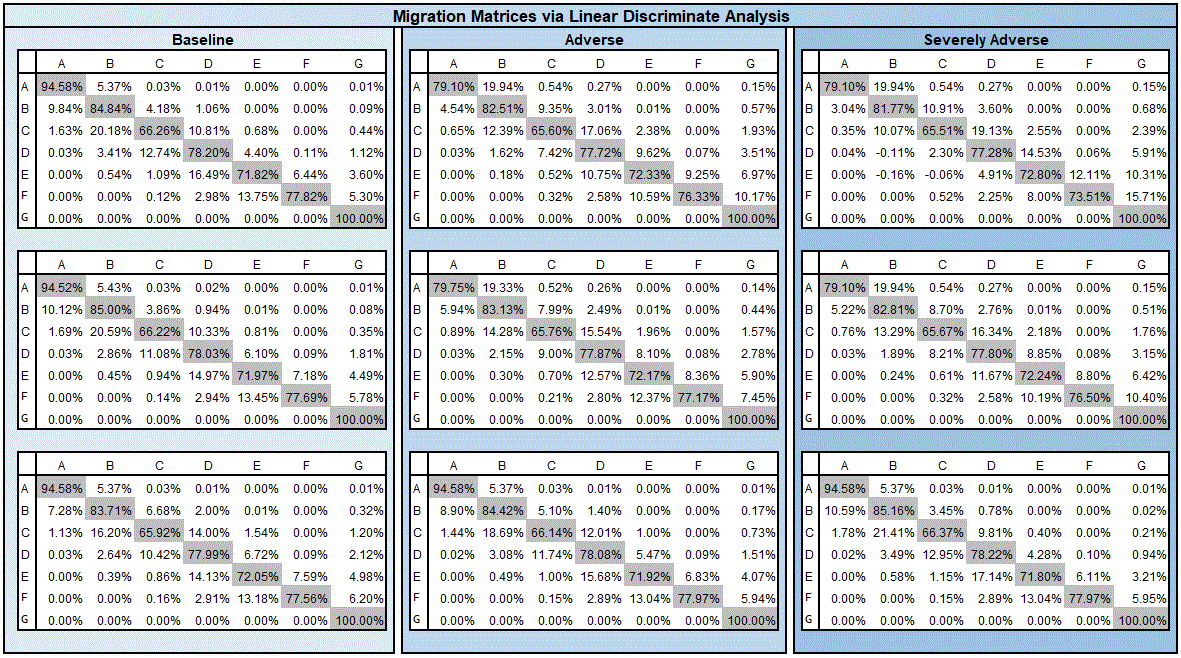# Machine Learning and Credit Risk (part 6) – Multi-class Linear Discriminate Analysis

Linear Discriminate Analysis (LDA) is another method which should be familiar to statisticians and economists.  LDA is a dimensionality reduction technique which has found its use in machine learning because of how well it functions as a classifier. Its primary goal is to project data onto a lower dimensional space.

A basic principal is to maximize the difference between the means of two groups, while minimizing the variance among members of the same group.

Although LDA and Principal Component Analysis are both primarily dimensionality reduction techniques, LDA differs from PCA in because in addition to finding the component axises with LDA we are interested in the axes that maximize the separation between multiple classes.

LDA has strong roots in credit risk. Probability the most famous credit scoring model (Altman’s Z-score) was derived from Linear Discriminate Analysis. The Altman’s Z score is mapped to a credit rating to produce the relevant probability of default for borrower.

For our purposes we use a Multi-class LDA. Multi-class LDA is a generalization of standard two-class LDA that can handle more than two classes.

• The LDA fails when the discriminatory information is not in the mean but instead in the variance
• Classes are Unimodal Gaussians. DO not work well with non-Gaussian distributions
• There are newer algorithms that have been shown t

Figure 10 displays the conditional matrices derived by the Linear Discriminate Analysis approach. It displays the forecasted migration matrices conditioned on Baseline, Adverse, and Severely Adverse economic conditions.```library("RTransProb")

for (i in c(24, 25, 26)) {
data &lt;- data

histData &lt;- histData.normz

predData_lda2 &lt;- predData_lda
predData_lda2 &lt;- subset(
predData_lda2,
X == i,
select = c(Market.Volatility.Index..Level..normz
)
)

indVars   = c("Market.Volatility.Index..Level..normz"
)

startDate = "1991-08-16"
endDate   = "2007-08-16"

depVar &lt;- c("end_rating")
pct &lt;- 1
wgt &lt;-  "mCount"
ratingCat &lt;- c("A", "B", "C", "D", "E", "F", "G")
defind    &lt;- "G"
method    = "cohort"
snapshots = 1
interval  = 1

lda_TM &lt;-
transForecast_lda(
data, histData, predData_lda2, startDate, endDate, method,
interval, snapshots, defind, depVar, indVars, pct, ratingCat
)
print(lda_TM)

}

```

1.shilkaren says: